﻿ 基于低秩分解的鲁棒典型相关分析
«上一篇文章快速检索 高级检索

 智能系统学报2017, Vol. 12Issue (4): 491-497  DOI: 10.11992/tis.2016070240

### 引用本文NI Huaifa, SHEN Xiaobo, SUN Quansen. Robust canonical correlation analysis based onlow rank decomposition[J]. CAAI Transactions on Intelligent Systems, 2017, 12(4): 491-497. DOI: 10.11992/tis.201607024.### 文章历史

Robust canonical correlation analysis based onlow rank decomposition
NI Huaifa, SHEN Xiaobo, SUN QuansenSchool of Computer Science and Engineering, Nanjing University of Science and Technology, Nanjing 210094, China
Abstract: Canonical correlation analysis (CCA) is a popular multi-feature extraction method, which can effectively explore the correlations between two sets of features. Up to now, CCA has been widely used in pattern recognition, however it has limited feature extraction power for large noisy data. For CCA to deal better with noisy data, a new method, robust canonical correlation analysis (RbCCA), based on low rank decomposition, is proposed. RbCCA first decomposes features using low rank decomposition to get the low rank and noisy components, then it constructs new covariance matrices based on these two components. A discriminative criteria function is further established to obtain discriminative projections by maximizing the correlations of the low rank component and minimizing the correlations of the noisy component. Experimental results on a MFEAT handwritten dataset, and ORL and Yale face datasets show that RbCCA can achieve higher recognition rates than existing CCA methods, especially in noisy settings.
Key words: Pattern recognition    feature extraction    data dimensionality reduction    canonical correlation analysis    low rank representation    low rank decomposition    low rank component    noise component

1 典型相关分析基本原理

 $\begin{array}{*{20}{c}} {{\rho _{{\rm{CCA}}}}\left( {\mathit{\boldsymbol{\alpha }},\mathit{\boldsymbol{\beta }}} \right) = \frac{{\mathit{\boldsymbol{E}}\left( {{\mathit{\boldsymbol{\alpha }}^{\rm{T}}}\mathit{\boldsymbol{x}}{\mathit{\boldsymbol{y}}^{\rm{T}}}\mathit{\boldsymbol{\beta }}} \right)}}{{\sqrt {\mathit{\boldsymbol{E}}\left( {{\mathit{\boldsymbol{\alpha }}^{\rm{T}}}\mathit{\boldsymbol{x}}{\mathit{\boldsymbol{x}}^{\rm{T}}}\mathit{\boldsymbol{\alpha }}} \right) \cdot \mathit{\boldsymbol{E}}\left( {{\mathit{\boldsymbol{\beta }}^{\rm{T}}}\mathit{\boldsymbol{y}}{\mathit{\boldsymbol{y}}^{\rm{T}}}\mathit{\boldsymbol{\beta }}} \right)} }} = }\\ {\frac{{{\mathit{\boldsymbol{\alpha }}^{\rm{T}}}{\mathit{\boldsymbol{S}}_{xy}}\mathit{\boldsymbol{\beta }}}}{{\sqrt {{\mathit{\boldsymbol{\alpha }}^{\rm{T}}}{\mathit{\boldsymbol{S}}_{xx}}\mathit{\boldsymbol{\alpha }} \times {\mathit{\boldsymbol{\alpha }}^{\rm{T}}}{\mathit{\boldsymbol{S}}_{yy}}\mathit{\boldsymbol{\beta }}} }}} \end{array}$ (1)

 ${\mathit{\boldsymbol{\alpha }}^{\rm{T}}}{\mathit{\boldsymbol{S}}_{xx}}\mathit{\boldsymbol{\alpha }} = {\mathit{\boldsymbol{\beta }}^{\rm{T}}}{\mathit{\boldsymbol{S}}_{yy}}\mathit{\boldsymbol{\beta }} = 1$ (2)

 $\begin{array}{l} \mathop {\max }\limits_{\mathit{\boldsymbol{\alpha }},\mathit{\boldsymbol{\beta }}} {\mathit{\boldsymbol{\rho }}_{{\rm{CCA}}}}\left( {\mathit{\boldsymbol{\alpha }},\mathit{\boldsymbol{\beta }}} \right) = {\mathit{\boldsymbol{\alpha }}^{\rm{T}}}{\mathit{\boldsymbol{S}}_{xy}}\mathit{\boldsymbol{\beta }}\\ {\rm{s}}{\rm{.t}}{\rm{.}}\;\;\;\;{\mathit{\boldsymbol{\alpha }}^{\rm{T}}}{\mathit{\boldsymbol{S}}_{xx}}\mathit{\boldsymbol{\alpha = }}{\mathit{\boldsymbol{\beta }}^{\rm{T}}}{\mathit{\boldsymbol{S}}_{yy}}\mathit{\boldsymbol{\beta = }}1 \end{array}$ (3)

2 基于低秩分解的鲁棒典型相关分析

2.1 RbCCA协方差矩阵的构建

 $\begin{array}{*{20}{c}} {\mathop {\min }\limits_{Z,E} {{\left\| Z \right\|}_ * } + \lambda {{\left\| E \right\|}_{{l_1}}}}\\ {{\rm{s}}{\rm{.t}}{\rm{.}}\;\;\;\mathit{\boldsymbol{W}} = \mathit{\boldsymbol{Z}} + \mathit{\boldsymbol{E}}} \end{array}$ (4)

 $\left\{ \begin{array}{l} \mathit{\boldsymbol{S}}_{xx}^Z = E\left[ {{{\left( {\mathit{\boldsymbol{x}}_Z^N - E\left( {\mathit{\boldsymbol{x}}_Z^N} \right)} \right)}^{\rm{T}}}\left( {\mathit{\boldsymbol{x}}_Z^N - E\left( {\mathit{\boldsymbol{x}}_Z^N} \right)} \right)} \right]\\ \mathit{\boldsymbol{S}}_{xx}^E = E\left[ {{{\left( {\mathit{\boldsymbol{x}}_E^N - E\left( {\mathit{\boldsymbol{x}}_E^N} \right)} \right)}^{\rm{T}}}\left( {\mathit{\boldsymbol{x}}_E^N - E\left( {\mathit{\boldsymbol{x}}_E^N} \right)} \right)} \right]\\ \mathit{\boldsymbol{S}}_{xy}^Z = E\left[ {{{\left( {\mathit{\boldsymbol{x}}_Z^N - E\left( {\mathit{\boldsymbol{x}}_Z^N} \right)} \right)}^{\rm{T}}}\left( {\mathit{\boldsymbol{y}}_Z^N - E\left( {\mathit{\boldsymbol{y}}_Z^N} \right)} \right)} \right]\\ \mathit{\boldsymbol{S}}_{xy}^E = E\left[ {{{\left( {\mathit{\boldsymbol{x}}_E^N - E\left( {\mathit{\boldsymbol{x}}_E^N} \right)} \right)}^{\rm{T}}}\left( {\mathit{\boldsymbol{y}}_E^N - E\left( {\mathit{\boldsymbol{x}}_E^N} \right)} \right)} \right] \end{array} \right.$

2.2 算法原理与模型构建

RbCCA将xNyN的低秩分量，噪声分量区别对待，即求一对投影方向αβ，使得αTSxyZβ尽可能大的同时αTSxyEβ尽可能小。于是得到改进后的典型相关准则函数为

 $\left\{ \begin{array}{l} \mathop {\max }\limits_{\alpha ,\beta } \mathit{\boldsymbol{\rho }}_{{\rm{CCA}}}^Z\left( {\mathit{\boldsymbol{\alpha }},\mathit{\boldsymbol{\beta }}} \right) = {\mathit{\boldsymbol{\alpha }}^{\rm{T}}}\mathit{\boldsymbol{S}}_{xy}^Z\mathit{\boldsymbol{\beta }}\\ \mathop {\min }\limits_{\alpha ,\beta } \mathit{\boldsymbol{\rho }}_{{\rm{CCA}}}^E\left( {\mathit{\boldsymbol{\alpha }},\mathit{\boldsymbol{\beta }}} \right) = {\mathit{\boldsymbol{\alpha }}^{\rm{T}}}\mathit{\boldsymbol{S}}_{xy}^E\mathit{\boldsymbol{\beta }} \end{array} \right.$ (5)

 $\begin{array}{*{20}{c}} {\mathop {\max }\limits_{\alpha ,\beta } \left\{ {\mathit{\boldsymbol{\rho }}_{{\rm{CCA}}}^Z\left( {\mathit{\boldsymbol{\alpha }},\mathit{\boldsymbol{\beta }}} \right) - \mathit{\boldsymbol{\rho }}_{{\rm{CCA}}}^E\left( {\mathit{\boldsymbol{\alpha }},\mathit{\boldsymbol{\beta }}} \right)} \right\} = }\\ {{\mathit{\boldsymbol{\alpha }}^{\rm{T}}}\mathit{\boldsymbol{S}}_{xy}^Z\mathit{\boldsymbol{\beta }} - {\mathit{\boldsymbol{\alpha }}^{\rm{T}}}\mathit{\boldsymbol{S}}_{xy}^E\mathit{\boldsymbol{\beta }} = }\\ {{\mathit{\boldsymbol{\alpha }}^{\rm{T}}}\left( {{\mathit{\boldsymbol{\alpha }}^{\rm{T}}}\mathit{\boldsymbol{S}}_{xy}^Z\mathit{\boldsymbol{\beta }} - \mathit{\boldsymbol{S}}_{xy}^E} \right)\mathit{\boldsymbol{\beta }}} \end{array}$ (6)

 $\left\{ \begin{array}{l} {\mathit{\boldsymbol{\alpha }}^{\rm{T}}}\left( {\mathit{\boldsymbol{S}}_{xx}^Z - \mathit{\boldsymbol{S}}_{xx}^E} \right)\mathit{\boldsymbol{\alpha = }}1\\ {\mathit{\boldsymbol{\beta }}^{\rm{T}}}\left( {\mathit{\boldsymbol{S}}_{yy}^Z - \mathit{\boldsymbol{S}}_{yy}^E} \right)\mathit{\boldsymbol{\beta = }}1 \end{array} \right.$ (7)

Sxy*替代式(7) 中的SxyZSxyESxx*Syy*分别替代式(7) 中的SxxZ-SxxESyyZ-SyyE，综合式(6)、(7)，得到转换后的RbCCA的准则函数：

 $\begin{array}{*{20}{c}} {\mathop {\max }\limits_{\mathit{\boldsymbol{\alpha }},\mathit{\boldsymbol{\beta }}} \mathit{\boldsymbol{\rho }}_{{\rm{CCA}}}^ * \left( {\mathit{\boldsymbol{\alpha }},\mathit{\boldsymbol{\beta }}} \right) = {\mathit{\boldsymbol{\alpha }}^{\rm{T}}}\mathit{\boldsymbol{S}}_{xy}^ * \mathit{\boldsymbol{\beta }}}\\ {{\rm{s}}{\rm{.t}}{\rm{.}}\;\;{\mathit{\boldsymbol{\alpha }}^{\rm{T}}}\mathit{\boldsymbol{S}}_{xx}^ * \mathit{\boldsymbol{\alpha }} = 1,{\mathit{\boldsymbol{\beta }}^{\rm{T}}}\mathit{\boldsymbol{S}}_{yy}^ * \mathit{\boldsymbol{\beta }} = 1} \end{array}$ (8)

2.3 RbCCA模型求解

 $\left\{ \begin{array}{l} \mathit{\boldsymbol{S}}_{xy}^ * {\left( {\mathit{\boldsymbol{S}}_{yy}^ * } \right)^{ - 1}}\mathit{\boldsymbol{S}}_{yx}^ * \mathit{\boldsymbol{\alpha = }}{\zeta ^2}\mathit{\boldsymbol{S}}_{xx}^ * \mathit{\boldsymbol{\alpha }}\\ \mathit{\boldsymbol{S}}_{yx}^ * {\left( {\mathit{\boldsymbol{S}}_{xx}^ * } \right)^{ - 1}}\mathit{\boldsymbol{S}}_{xy}^ * \mathit{\boldsymbol{\beta = }}{\zeta ^2}\mathit{\boldsymbol{S}}_{yy}^ * \mathit{\boldsymbol{\beta }} \end{array} \right.$ (9)

 $P = \left[ {\begin{array}{*{20}{c}} {{{\left( {{\mathit{\boldsymbol{\alpha }}^ * }} \right)}^{\rm{T}}}\mathit{\boldsymbol{x}}}&{{{\left( {{\mathit{\boldsymbol{\beta }}^ * }} \right)}^{\rm{T}}}\mathit{\boldsymbol{y}}} \end{array}} \right]$ (10)

RbCCA既满足了多表示数据的维数约减要求，保持了较好的识别率，又填补了传统CCA在噪声数据下特征表示能力差的缺陷，提升了CCA处理噪声数据的能力。

3 实验结果与分析

3.1 MFEAT手写体数据库

MFEAT手写体数据库一共包含fac、fou、kar、mor、pix、zer 6组特征，每组特征包含0~9共10个数字，每个数字类别包含200个样本。6组特征，每两组特征进行组合共15种组合，随机挑选其中的10种组合做10次随机实验，每次实验从每种组合中随机选择100个作为训练样本，100个作为测试样本。训练样本总数和测试样本总数均为1 000。实验开始前，在原始MFEAT数据库上，分别在6组特征加入10%的椒盐噪声来模拟实际应用中的噪声。表 1 MFEAT不同特征组合下识别算法识别率 Tab.1 Different characteristics of the combination identify the algorithm recognition rate in MFEAT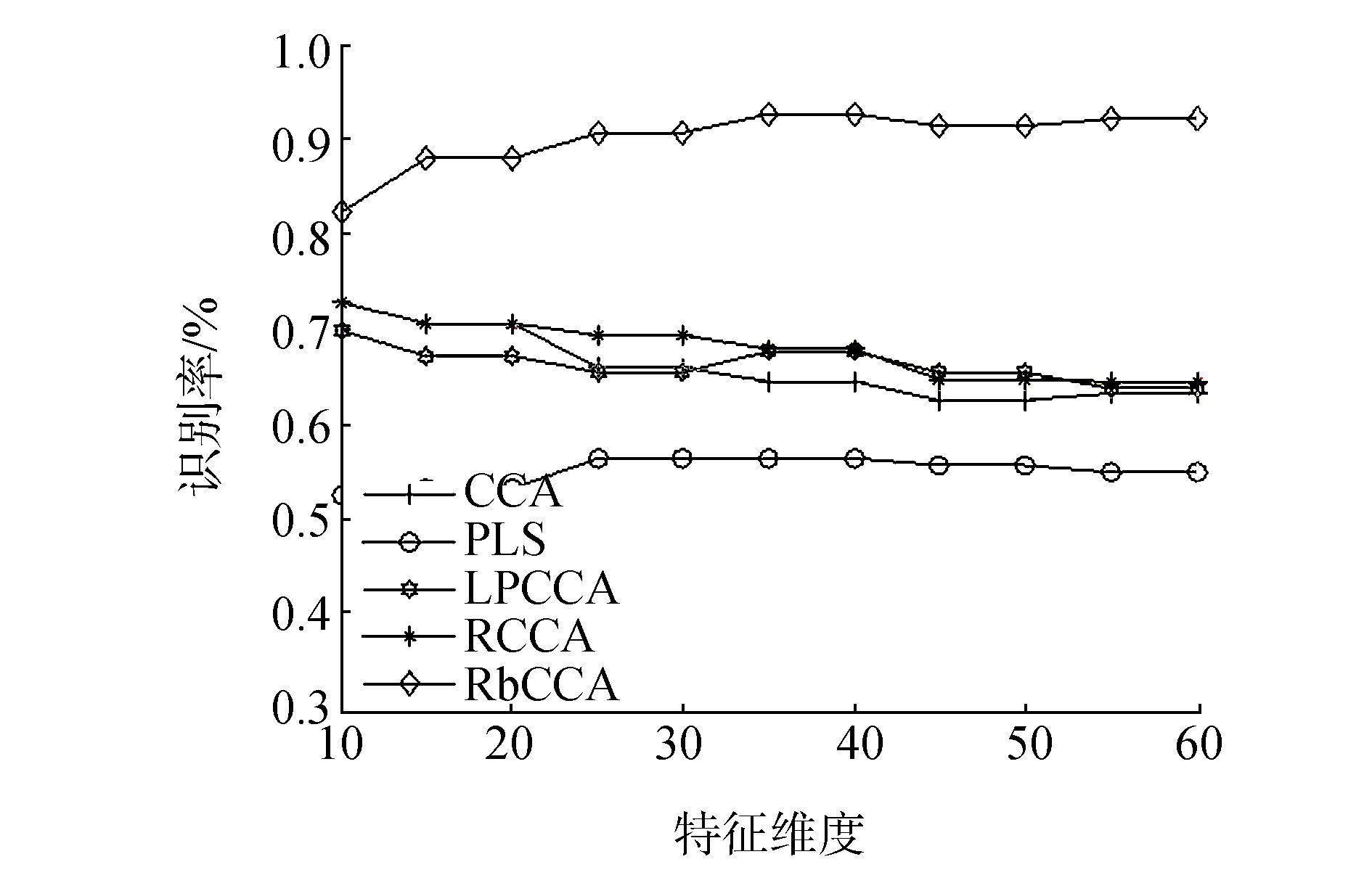图 1 识别方法识别结果随着特征维度增加的识别曲线图 Fig.1 The recognition curve of the recognition method with the increase of the feature dimension
3.2 ORL人脸数据库

ORL人脸数据库由剑桥大学AT & T实验室创建，包含40人共400张面部图像，每幅图像大小92×112像素，部分图像包括了姿态，表情和面部饰物的变化。图 2给出了ORL人脸库中某人的10张图像。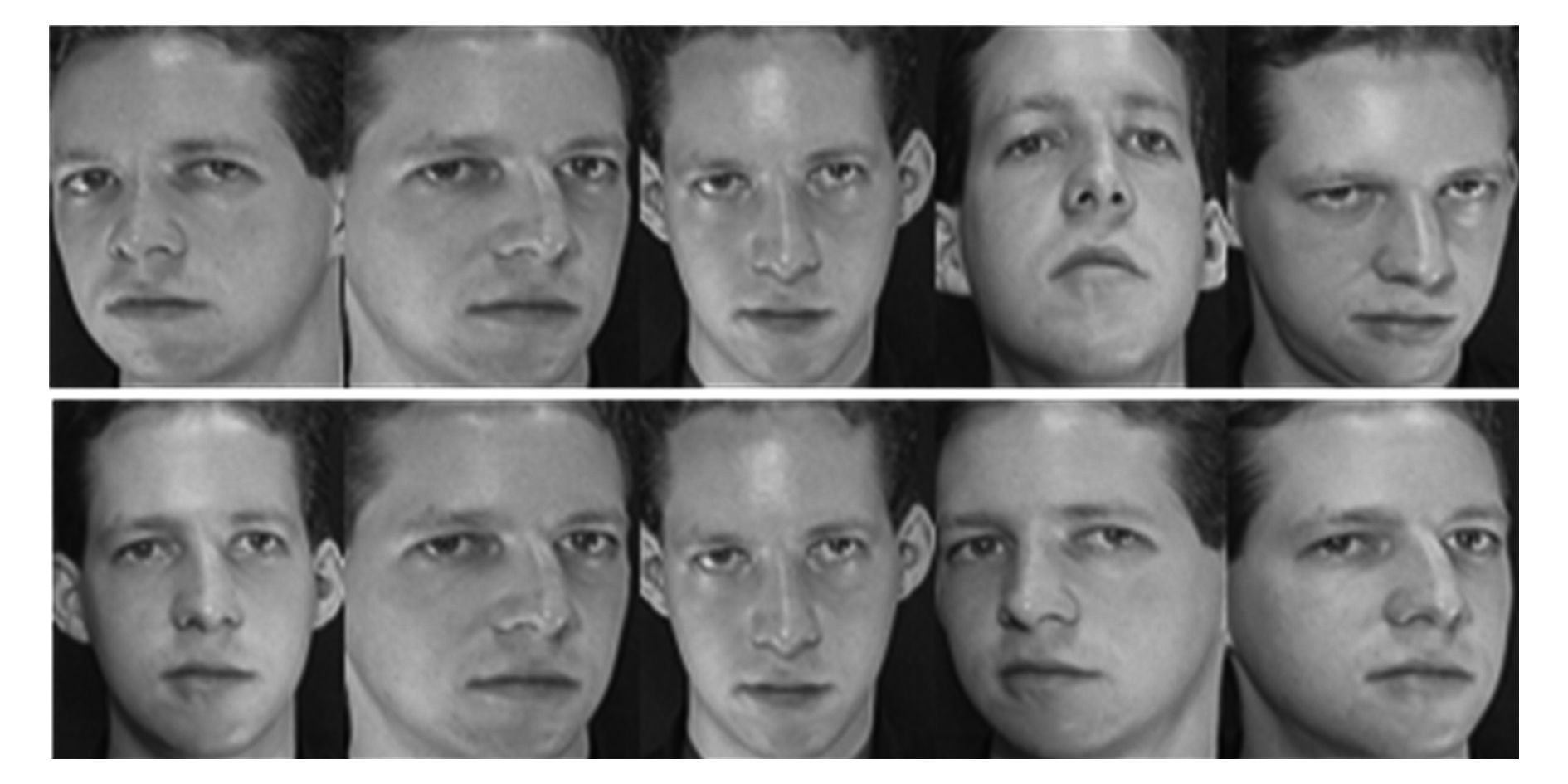图 2 ORL人脸数据库中某人的10张图像 Fig.2 10 images of a person in the ORL face database表 2 ORL人脸库不同的训练样本数下识别算法的识别率 Tab.2 ORL face database under different training samples identify the recognition rate of the algorithm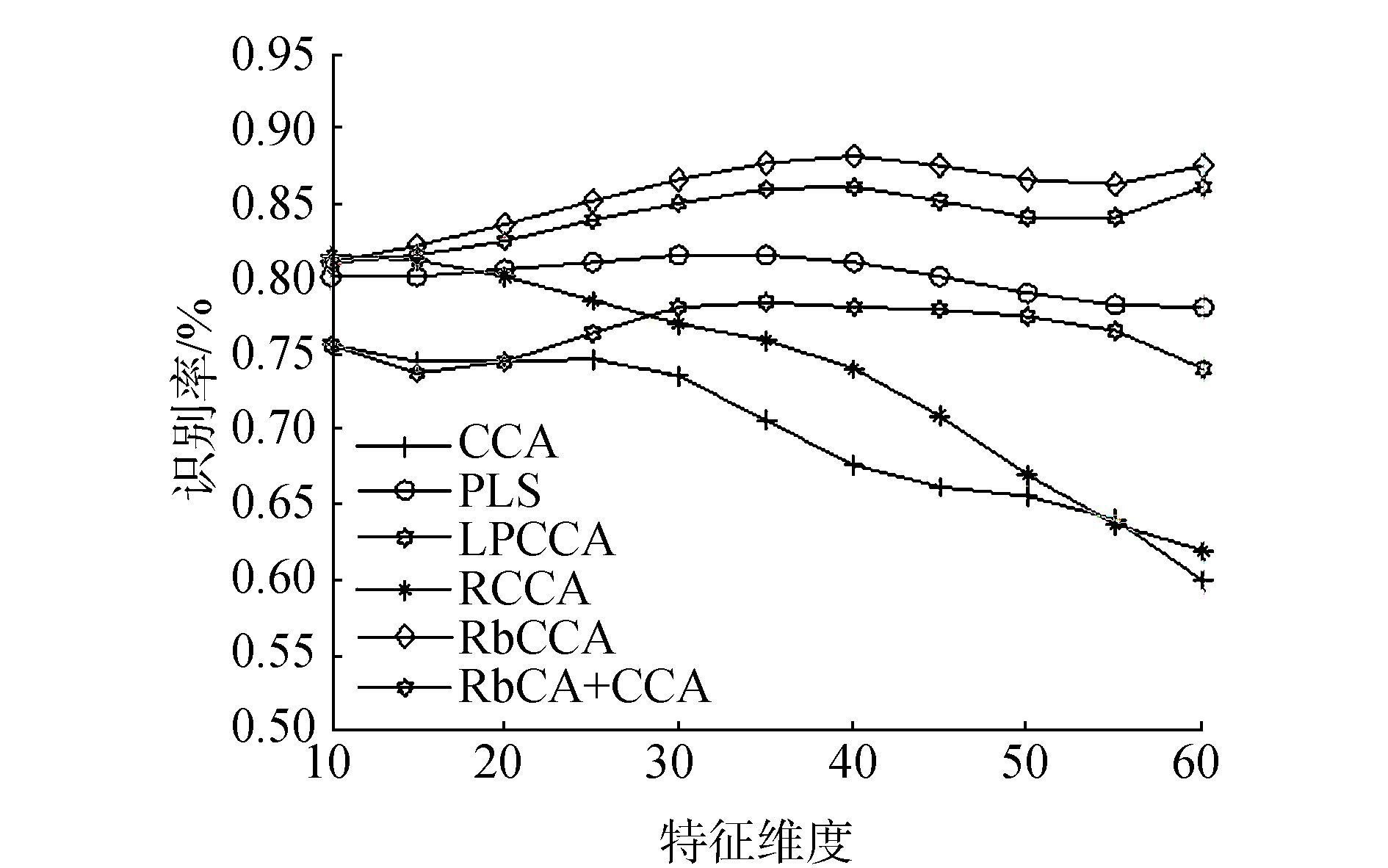图 3 识别方法识别结果随特征维度增加的变化曲线图 Fig.3 The recognition curve of the recognition method with the increase of the feature dimension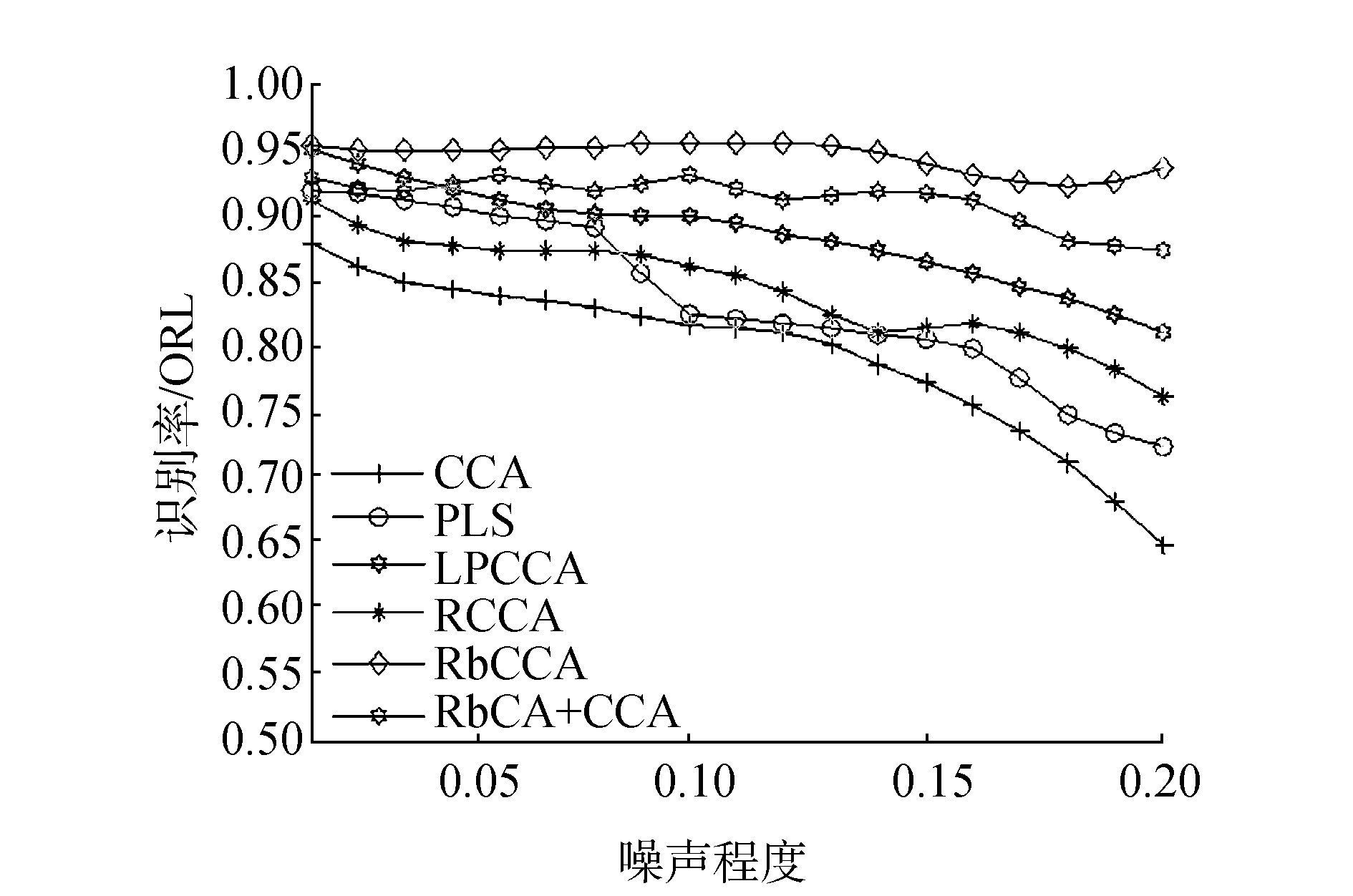图 4 识别方法识别结果随着噪声强度增加的变化曲线图 Fig.4 The result of the recognition method with the increase of the noise intensity
3.3 Yale数据库实验

Yale人脸数据库[18-21]包含15个人的165幅灰度图像，每人11幅，包括光照方向(左、右和正面)、眼睛、表情变化(正常、愉快、悲伤、困乏、惊讶和眨眼)。图像大小为100像素×100像素。图 5是Yale人脸库中某人的11幅图像。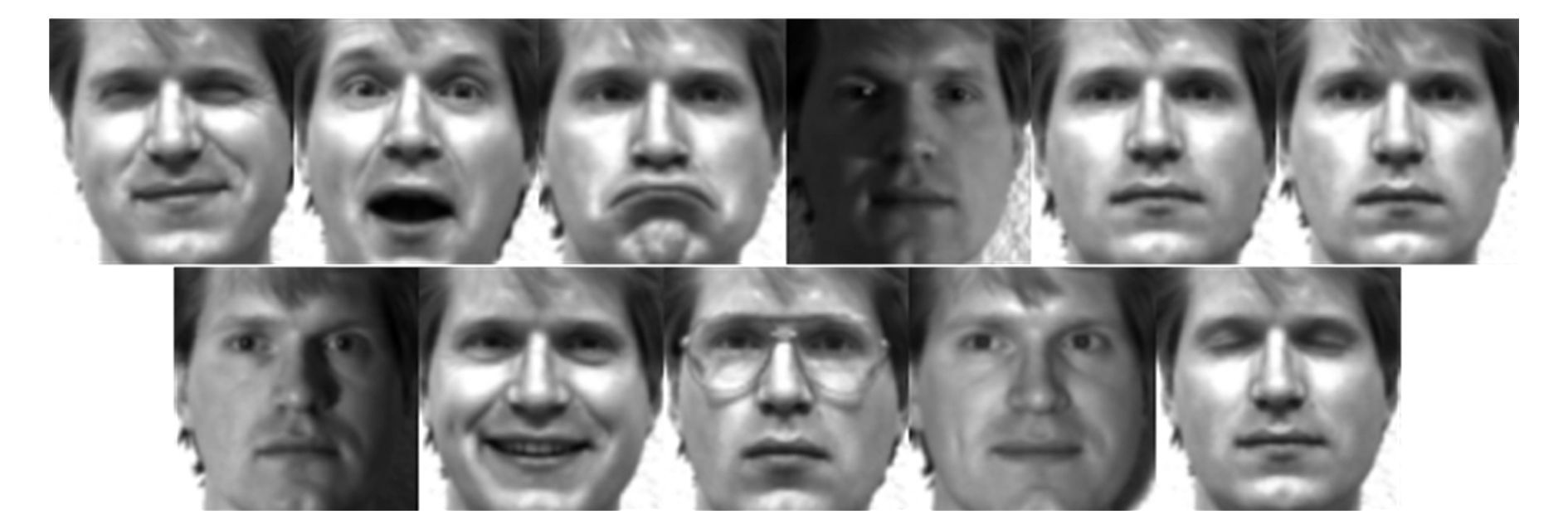图 5 Yale数据库中某人的11幅图像 Fig.5 11 images of one person in Yale database表 3 Yale人脸库不同的训练样本数下，CCA、PLS、LPCCA、RCCA、RPCA+CCA、RbCCA算法的识别率 Tab.3 Recognition rates for CCA, PLS, LPCCA, RCCA, RPCA + CCA, RbCCA algorithms for different training samples in the Yale face database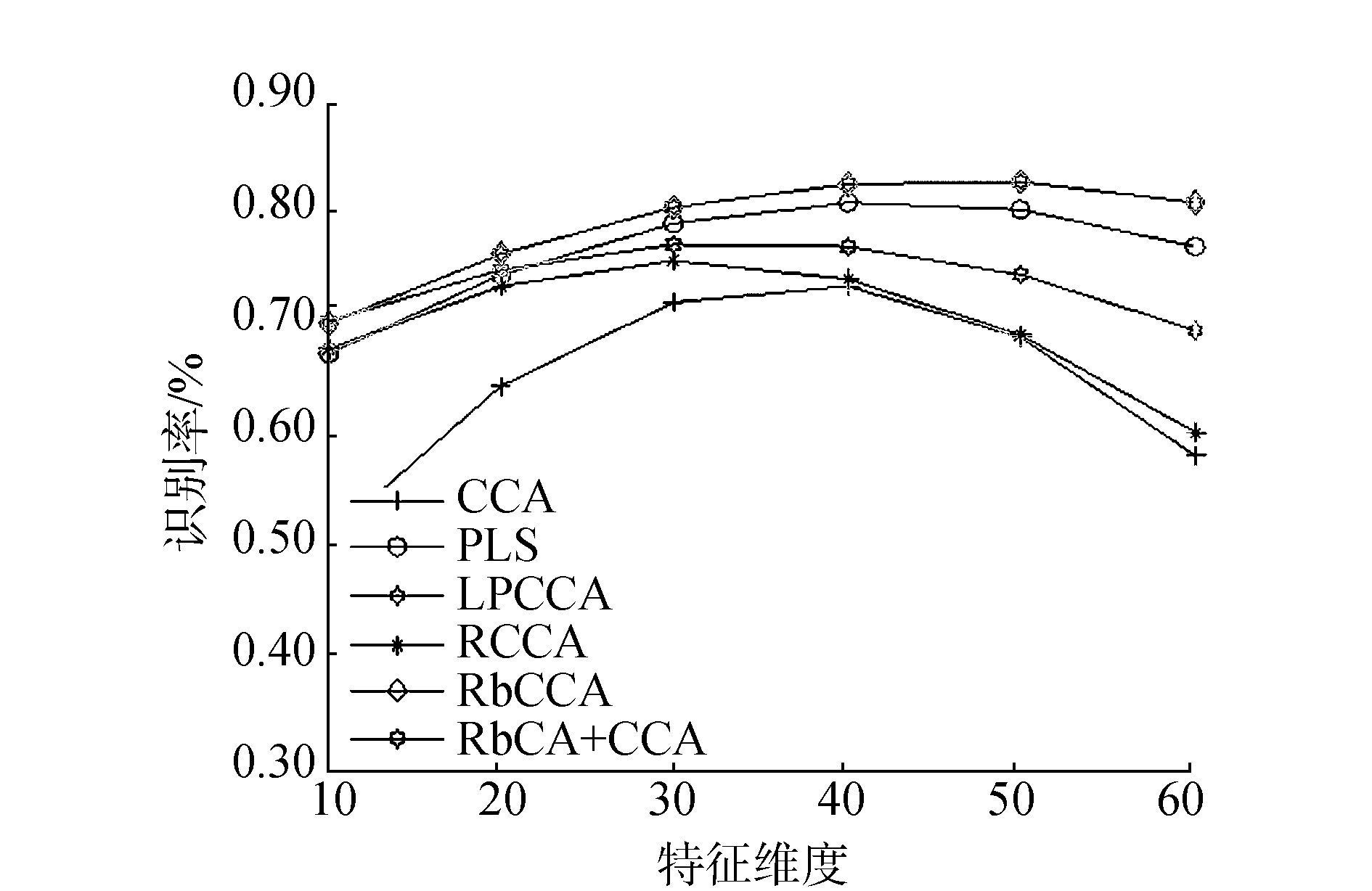图 6 识别方法识别结果随着特征维度增加的变化曲线图 Fig.6 The recognition curve of the recognition method with the increase of the feature dimension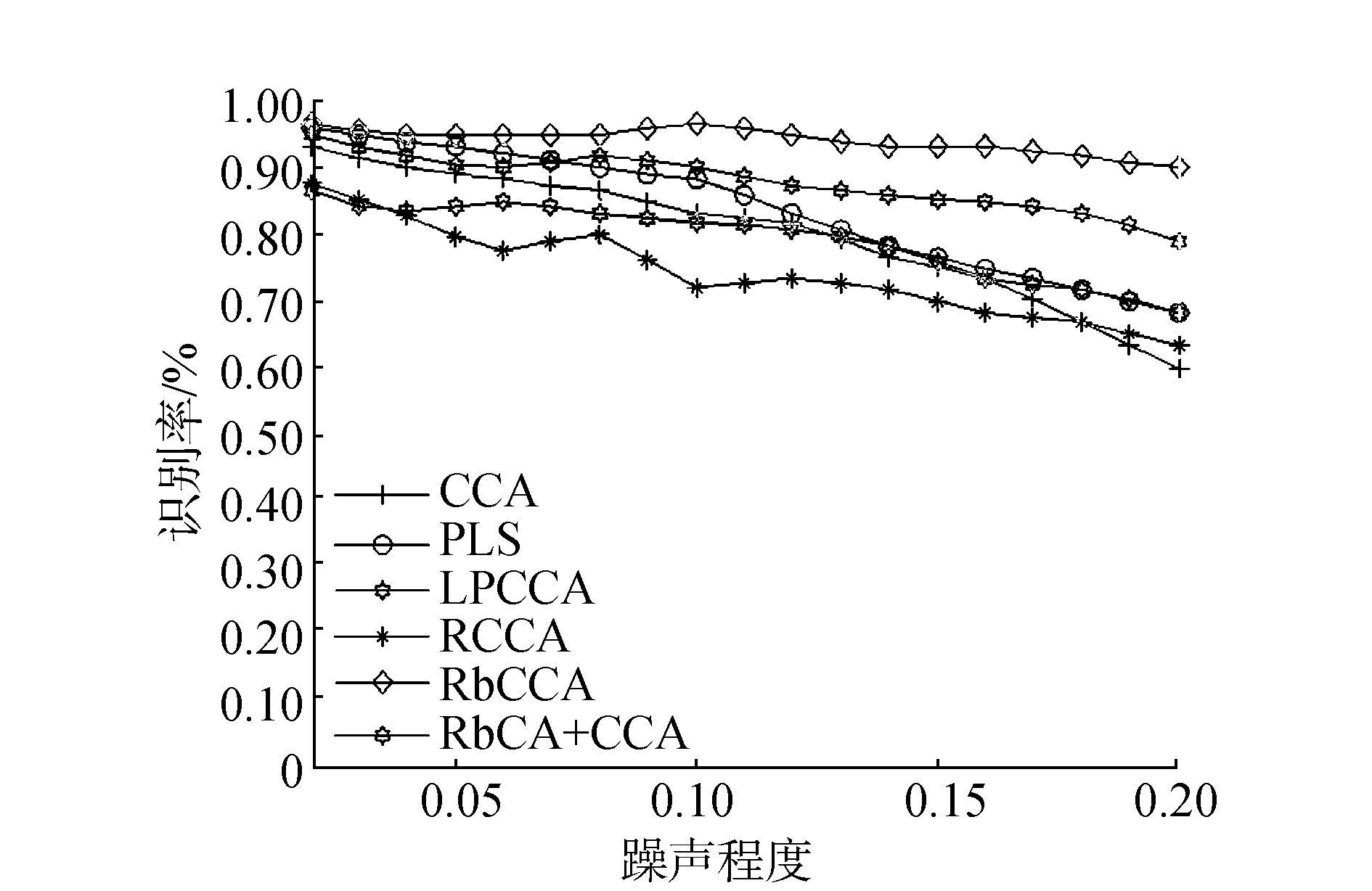图 7 识别方法识别结果随着噪声强度增加的变化曲线图 Fig.7 The result of the recognition method with the increase of the noise intensity
4 结束语

  FUKUNAGA K. Introduction to statistical pattern recognition[M]. Academic press, 2013. (0)  FISHER R A. The use of multiple measurements in axonomic problems[J]. Annals of eugenics, 1936, 7(2): 179-188. DOI:10.1111/j.1469-1809.1936.tb02137.x (0)  杨勇, 蔡舒博. 一种基于两步降维和并行特征融合的表情识别方法[J]. 重庆邮电大学学报:自然科学版, 2015, 27(3): 377-387. YANG Yong, CAI Shubo. Facial expression recognition method based on two-steps dimensionality reduction and parallel feature fusion[J]. Journal of chongqing university of posts and telecommunications:natural science edition, 2015, 27(3): 377-387. (0)  HOU C, ZHANG C, WU Y, et al. Multiple view semi-supervised dimensionality reduction[J]. Pattern recognition, 2010, 43(3): 720-730. DOI:10.1016/j.patcog.2009.07.015 (0)  HOTELLING H. Relations between two sets of variates[J]. Biometrika, 1936, 28(3/4): 321-377. DOI:10.2307/2333955 (0)  孙权森, 基于相关投影分析的特征抽取与图像识别研究[D]. 南京: 南京理工大学, 2006. SUN Quansen. Research on feature extraction and image recognition based on correlation projection analysis[D]. Nanjing:Nanjing University of Science and Technology, 2006. http://cdmd.cnki.com.cn/Article/CDMD-10288-2006183401.htm (0)  HOUShudong, 孙权森. 稀疏保持典型相关分析及在特征融合中的应用[J]. 自动化学报, 2012, 38(4): 659-665. HOU Shudong, SUN Quansen. Sparse maintaining canonical correlation analysis and its application in feature fusion[J]. Journal of automation, 2012, 38(4): 659-665. (0)  LIU G, LIN Z, YAN S, et al. Robust recovery of subspace structures by low-rank representation[J]. IEEE transactions on pattern analysis and machine intelligence, 2013, 35(1): 171-184. DOI:10.1109/TPAMI.2012.88 (0)  WRIGHT J, GANESH A, RAO S, et al. Robust principal component analysis:Exact recovery of corrupted low-rank matrices via convex optimization[C]//Advances in neural information processing systems. Vancouver, BC, Canada, 2009:2080-2088. (0)  LIU G, LIN Z, YU Y. Robust subspace segmentation by low-rank representation[C]//Proceedings of the 27th international conference on machine learning (ICML-10). Haifa, Israel. 2010:663-670. (0)  袁运浩. 多重集典型相关分析理论及在高维多表示数据中的应用[D]. 南京: 南京理工大学, 2013. YUAN Yunhao. Canonical correlation analysis theory of multiple sets and its application in multi-representation data[D]. Nanjing:Nanjing University of Science and Technology, 2013. (0)  孙权森, 曾生根, 王平安, 等. 典型相关分析的理论及其在特征融合中的应用[J]. 计算机学报, 2005, 28(9): 1524-1533. SUN Quansen, ZENG Shenggen, WANG Pingan, et al. The theory of canonical correlation analysis and its application in feature fusion[J]. Chinese journal of computers, 2005, 28(9): 1524-1533. (0)  张凡龙. 基于核范数的低秩理论与方法研究[D]. 南京: 南京理工大学, 2015. ZHANG Fanlong. Research on low rank theory and method based on kernel norm[D]. Nanjing:Nanjing University of Science and Technology, 2015. (0)  CANDÈS E J, LI X, MA Y, et al. Robust principal component analysis[J]. Journal of the ACM (JACM), 2011, 58(3): 11. (0)  BAO B K, LIU G, XU C, et al. Inductive robust principal component analysis[J]. IEEE transactions on image processing, 2012, 21(8): 3794-3800. DOI:10.1109/TIP.2012.2192742 (0)  SHEN X B, SUN Q S, YUAN Y H. A unified multiset canonical correlation analysis framework based on graph embedding for multiple feature extraction[J]. Neurocomputing, 2015, 148: 397-408. DOI:10.1016/j.neucom.2014.06.015 (0)  FERDINANDO S, ANDY H. Parameterisation of a stochastic model for human face identification[C]//Proceedings of 2nd IEEE Workshop on Applications of Computer Vision. Sarasota FL, 1994. (0)  BELHUMEUR P, HEPANHA J, KRIEGMAN D. Eigenfaces vs. Fisherfaces:recognition using class specific linear projection[J]. IEEE transactions on pattern analysis and machine intelligence, 1997, 19(7): 711-720. DOI:10.1109/34.598228 (0)  袁宝华, 王欢, 任明武. 基于完整LBP特征的人脸识别[J]. 计算机应用研究, 2012, 29(4): 1557-1559. YUAN Baohua, WANG Huan, REN Mingwu. Face recognition based on complete lbp feature[J]. Journal of computer applications, 2012, 29(4): 1557-1559. (0)  何国辉, 甘俊英. 二维主元分析在人脸识别中的应用研究[J]. 计算机工程与设计, 2006, 27(24): 4667-4669. HE Guohui, GAN Junying. Application of two-dimensional principal component analysis in face recognition[J]. Computer engineering and design, 2006, 27(24): 4667-4669. DOI:10.3969/j.issn.1000-7024.2006.24.018 (0)  赵军, 赵艳, 杨勇, 等. 基于降维的堆积降噪自动编码机的表情识别方法[J]. 重庆邮电大学学报:自然科学版, 2016, 28(6): 844-848. ZHAO Jun, ZHAO Yan, YANG Yong, et al. Facial expression recognition method based on stacked denoisingauto-encoders and feature reduction[J]. Journal of chongqing university of posts and telecommunications:natural science edition, 2016, 28(6): 844-848. (0)